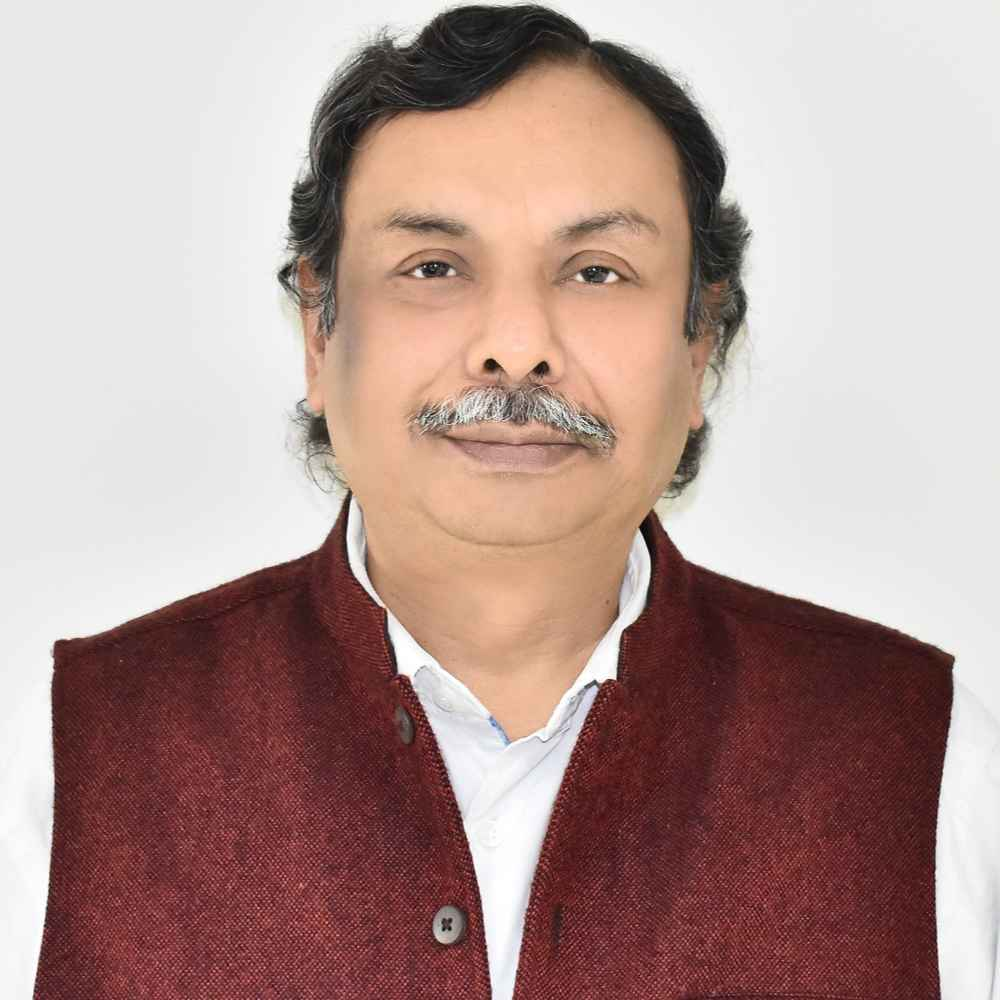X
Automated conversion of 2D to 3D image using manifold learning
N. Chahal, M. Pippal,
Published in Institute of Electrical and Electronics Engineers Inc.
2016
Abstract
Automatic technique of 2D to 3D image conversion is proposed using manifold learning and sequential labeling which generates very reliable and accurate 3D depth maps that are very close to ground truth depths. In paper, LLE which is a non linear and neighborhood preserving embedding algorithm is used for depth estimation of a 2D image. And then, fixed point supervised learning algorithm is applied to construct consistent and smooth 3D output. The high dimensional data points or pixels of the input frames can be represented by a linear combination of its nearest neighbors and a lower dimensional point is reconstructed while preserving the local and geometric properties of the frames. The neighbors are assigned to each input point in the image data set and their weight vectors are computed that best linearly reconstruct the input point from its neighbors. To get the depth value of input point in new image, the reconstruction weights of its closest neighbors in training samples are multiplied with their corresponding ground truth depth values. The fixed point learning algorithm takes depths from manifold and other image features as input vectors and generates more consistent and accurate depth images for better 3D conversion. © 2015 IEEE.
•